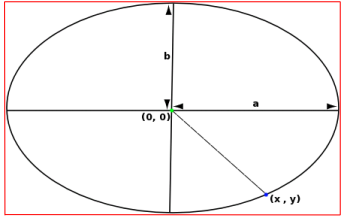# Class Ellipse2D

• `java.lang.Object`
• `java.awt.geom.RectangularShape`
• `java.awt.geom.Ellipse2D`
Implemented Interfaces:
Cloneable, Shape
Known Direct Subclasses:
Ellipse2D.Double, Ellipse2D.Float

`public abstract class Ellipse2D`
`extends RectangularShape`

Ellipse2D is the shape of an ellipse.The ellipse is defined by it's bounding box (shown in red), and is defined by the implicit curve:
(x/a)2 + (y/b)2 = 1

Since:
1.2

## Nested Class Summary

`static class`
`Ellipse2D.Double`
An `Ellipse2D` that stores its coordinates using `double` primitives.
`static class`
`Ellipse2D.Float`
An `Ellipse2D` that stores its coordinates using `float` primitives.

## Constructor Summary

`Ellipse2D()`
Ellipse2D is defined as abstract.

## Method Summary

` boolean`
`contains(double x, double y)`
Determines if a point is contained within the ellipse.
` boolean`
`contains(double x, double y, double w, double h)`
Determines if a rectangle is completely contained within the ellipse.
` PathIterator`
`getPathIterator(AffineTransform at)`
Returns a PathIterator object corresponding to the ellipse.

Note: An ellipse cannot be represented exactly in PathIterator segments, the outline is thefore approximated with cubic Bezier segments.

` boolean`
`intersects(double x, double y, double w, double h)`
Determines if a rectangle intersects any part of the ellipse.

### Methods inherited from class java.awt.geom.RectangularShape

`clone`, `contains`, `contains`, `getBounds`, `getCenterX`, `getCenterY`, `getFrame`, `getHeight`, `getMaxX`, `getMaxY`, `getMinX`, `getMinY`, `getPathIterator`, `getWidth`, `getX`, `getY`, `intersects`, `isEmpty`, `setFrame`, `setFrame`, `setFrame`, `setFrameFromCenter`, `setFrameFromCenter`, `setFrameFromDiagonal`, `setFrameFromDiagonal`

### Methods inherited from class java.lang.Object

`clone`, `equals`, `extends Object> getClass`, `finalize`, `hashCode`, `notify`, `notifyAll`, `toString`, `wait`, `wait`, `wait`

## Constructor Details

### Ellipse2D

`protected Ellipse2D()`
Ellipse2D is defined as abstract. Implementing classes are Ellipse2D.Float and Ellipse2D.Double.

## Method Details

### contains

```public boolean contains(double x,
double y)```
Determines if a point is contained within the ellipse.

Specified by:
contains in interface Shape
Parameters:
`x` - - x coordinate of the point.
`y` - - y coordinate of the point.
Returns:
true if the point is within the ellipse, false otherwise.

### contains

```public boolean contains(double x,
double y,
double w,
double h)```
Determines if a rectangle is completely contained within the ellipse.

Specified by:
contains in interface Shape
Parameters:
`x` - - x coordinate of the upper-left corner of the rectangle
`y` - - y coordinate of the upper-left corner of the rectangle
`w` - - width of the rectangle
`h` - - height of the rectangle
Returns:
true if the rectangle is completely contained, false otherwise.

### getPathIterator

`public PathIterator getPathIterator(AffineTransform at)`
Returns a PathIterator object corresponding to the ellipse.

Note: An ellipse cannot be represented exactly in PathIterator segments, the outline is thefore approximated with cubic Bezier segments.

Specified by:
getPathIterator in interface Shape
Parameters:
`at` - an optional transform.
Returns:
A path iterator.

### intersects

```public boolean intersects(double x,
double y,
double w,
double h)```
Determines if a rectangle intersects any part of the ellipse.

Specified by:
intersects in interface Shape
Parameters:
`x` - - x coordinate of the upper-left corner of the rectangle
`y` - - y coordinate of the upper-left corner of the rectangle
`w` - - width of the rectangle
`h` - - height of the rectangle
Returns:
true if the rectangle intersects the ellipse, false otherwise.